# HSSlive: Plus One & Plus Two Notes & Solutions for Kerala State Board

## BSEB Class 9 Maths Chapter 12 Heron’s Formula Ex 12.2 Textbook Solutions PDF: Download Bihar Board STD 9th Maths Chapter 12 Heron’s Formula Ex 12.2 Book AnswersBSEB Class 9 Maths Chapter 12 Heron’s Formula Ex 12.2 Textbook Solutions PDF: Download Bihar Board STD 9th Maths Chapter 12 Heron’s Formula Ex 12.2 Book Answers

BSEB Class 9th Maths Chapter 12 Heron’s Formula Ex 12.2 Textbooks Solutions and answers for students are now available in pdf format. Bihar Board Class 9th Maths Chapter 12 Heron’s Formula Ex 12.2 Book answers and solutions are one of the most important study materials for any student. The Bihar Board Class 9th Maths Chapter 12 Heron’s Formula Ex 12.2 books are published by the Bihar Board Publishers. These Bihar Board Class 9th Maths Chapter 12 Heron’s Formula Ex 12.2 textbooks are prepared by a group of expert faculty members. Students can download these BSEB STD 9th Maths Chapter 12 Heron’s Formula Ex 12.2 book solutions pdf online from this page.

## Bihar Board Class 9th Maths Chapter 12 Heron’s Formula Ex 12.2 Books Solutions

 Board BSEB Materials Textbook Solutions/Guide Format DOC/PDF Class 9th Subject Maths Chapter 12 Heron’s Formula Ex 12.2 Chapters All Provider Hsslive

## How to download Bihar Board Class 9th Maths Chapter 12 Heron’s Formula Ex 12.2 Textbook Solutions Answers PDF Online?

2. Click on the Bihar Board Class 9th Maths Chapter 12 Heron’s Formula Ex 12.2 Answers.
3. Look for your Bihar Board STD 9th Maths Chapter 12 Heron’s Formula Ex 12.2 Textbooks PDF.
4. Now download or read the Bihar Board Class 9th Maths Chapter 12 Heron’s Formula Ex 12.2 Textbook Solutions for PDF Free.

## BSEB Class 9th Maths Chapter 12 Heron’s Formula Ex 12.2 Textbooks Solutions with Answer PDF Download

Find below the list of all BSEB Class 9th Maths Chapter 12 Heron’s Formula Ex 12.2 Textbook Solutions for PDF’s for you to download and prepare for the upcoming exams:

## BSEB Bihar Board Class 9th Maths Solutions Chapter 12 Heron’s Formula Ex 12.2

Question 1.
A park, in the shape of a quadrilateral ABCD, has ∠C = 90°, AB = 9 m, BC = 12 m, CD = 5 m and AD = 8 m. How much area does it occupy?
Solution: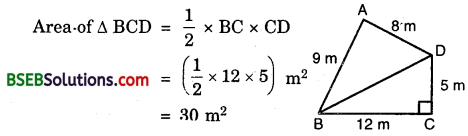Using Pythagoras theorem, we have BD² = BC² + BD²
⇒ BD² = 12² + 5²
⇒ BD² = 144 + 25
⇒ BD = 169‾‾‾‾√ = 13 m
For : ∆ ABD :
Let a = 13 m, b = 8 m and c = 9 m
s = 12(a + b + c)
= 12(13 + 8 + 9) m
= 12 x 30 m
= 15 m
s – a = (15 – 13) m = 2 m
s – b = (15 – 8) m = 7 m
s – c = (15 – 9) m = 6 m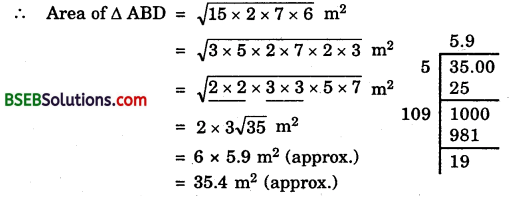∴ Required area = ∆ ABD + ∆ BCD
= 35.4 m² + 30 m²
= 65.4 m²

Question 2.
Find the area of a quadrilateral ABCD in which AB = 3 cm, BC = 4 cm, CD = 4 cm, DA = 5 cm and AC = 5 cm.
Solution:
Since AC² = AB² + BC²(as 5² = 3² + 4² i.e., 25 = 9 + 16)
∴ ∠ABC = 90°
Area of rt. ∠d ∆ ABC = 12 x AB x BC
= (12 x 3 x 4) cm² = 6 cm²
For ∆ ACD :
Let a = 5 cm, 6 = 4 cm and c = 5 cm. Then,
s = 12(a + b + c)
= 12(5 + 4 + 5) cm
= 12 x 14 cm
= 7 cm
Now, s – a = (7 – 5) cm = 2 cm
s – b = (7 – 4) cm = 3 cm
s – c = (7 – 5) cm = 2 cmArea of quadrilateral ABCD = Area of ∆ ABC + Area of ∆ ACD = (6 + 9.2) cm² = 15.2 cm² approx.

Question 3.
Radha made a picture of an aeroplane with coloured paper as shown in figure. Find the total area of the paper used.
Solution:
Paper used is of triangular shape. Let a = 15 m, b = 11 m and c = 6 m.Now, s = 12(a + b + c)
= 12(15 + 11 + 6)m
= 12 x 32 m = 16 m
= 12 x 32 m = 16 m
s – a = (16 – 15) m = 1 m
s – b = (16 – 11) m = 5 m
and,
s – c = (16 – 6) m = 10 m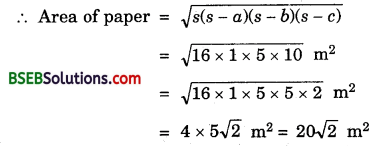Question 4.
A triangle and a parallelogram iiave the same base and the same area. If the sides of the triangle are 26 cm, 28 cm and 30 cm, and the parallelogram stands on the base 28 cm, find the height of the parallelogram.
Solution:
For the triangle :
Let its sides be a, b and c such that a = 26 cm, b = 28 cm and c = 30. Then,
s = 12(26 + 28 + 30) cm
= 12 x 84 cm = 42 cm
Now, s – a = (42 – 26) cm = 16 cm
s – b = (42 – 28) cm = 14 cm
and s – c = (42 – 30) cm = 12 cm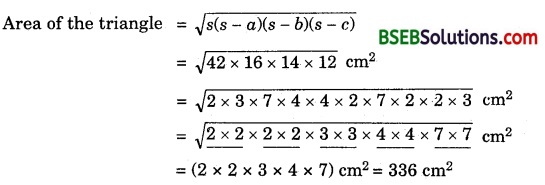For the parallelogram :
Area = base x heightQuestion 5.
A rhombus shaped field has green grass for 18 cows to graze. If each side of the rhombus is 30 m and its longer diagonal is 48 m, how much area of grass field will each cow be getting?
Solution:
We know that the diagonals of the rhombus bisect each other at right angles. Using Pythagoras theorem, we haveArea of one ∆ AOD = (12 x 24 x 18) m²
= 216 m²
∴ Area of rhombus = 4 x ∆ AOD
= (4 x 216) m²
= 864 m²
Grass area for 18 cows = 864 m²
Grass area for 1 cows = (86418) m² = 48 m²

Question 6.
An umbrella is made by stitching 10 triangular pieces of cloth of two different colours (See figure), each piece measuring 20 cm, 50 cm and 50 cm. How much cloth of each colour is required for the umbrella?Solution:
In one triangular piece, let a = 20 cm, b = 50 cm and c = 50 cm.
Now, s = 12(a + b + c) = 12 (20 + 50 + 50) cm
= 12 x 120 cm = 60 cm
s – a = (60 – 20) cm = 40 cm
s – b = (60 – 50) cm = 10 cm
and, s – c = (60 – 50) cm = 10 cm
∴ Area of one triangular piece
= 𝑠(𝑠−𝑎)(𝑠−𝑏)(𝑠−𝑐)‾‾‾‾‾‾‾‾‾‾‾‾‾‾‾‾‾‾‾‾√
= 60×40×10×10‾‾‾‾‾‾‾‾‾‾‾‾‾‾‾‾‾√ cm² = 2006‾√ cm²
∴ Area of 5 red triangles = 10006‾√cm²
and, area of 5 green triangles = 10006‾√ cm²

Question 7.
A kite in the shape of a square with a diagonal 32 cm and an isosceles triangle of base 8 cm and sides 6 cm each is to be made of three different shades as shown in figure. JBEow much paper of each shade has been used in it?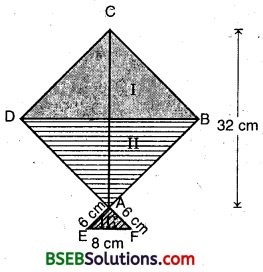Solution:
ABCD is a square such that AC = BC = 32 cm and AEF is an isosceles triangle in which AE = AF = 6 cm
For the area of shades I and II Clearly, from the figure
Area of shade I = Area of shade B = Area of Δ CDB
= 12 x DB x CM
= 12 x 32 x 16 sq cm
= 256 sq cm
For the area of shade III (i.e., A AEF)EL = LF = 12 EM
= 12 x 8 = 4 cm
and AE = 6 cm (given)
∴ AL = AE2−EL2‾‾‾‾‾‾‾‾‾‾‾√
= 36−16‾‾‾‾‾‾‾‾√ = 20‾‾‾√ = 25‾√ cm
Area of shade III = 12 x EF x AL
= 12 x x 25‾√ sq cm
= 85‾√ sq cm
= 8 x 2.24 sq cm (approx.)
= 17.92 sq cm (approx.)

Question 8.
A floral design on a floor is made up of 16 tiles which are triangular, the sides of the triangle being 9 cm, 28 cm and 35 cm (See figure). Find the cost of polishing the tiles at the rate of 50 p per cm².Solution:
For one triangular tile :
Let a = 9 cm, b = 28 cm and c = 35 cm
s = 12 (a + b + c) = 12(9 + 28+ 35) cm
= 12 x 72 cm = 36 cm
s – a = (36 – 9) cm = 27 cm
s – b = (36 – 28) cm = 8 cm
s – c = (36 – 35) cm = 1 cm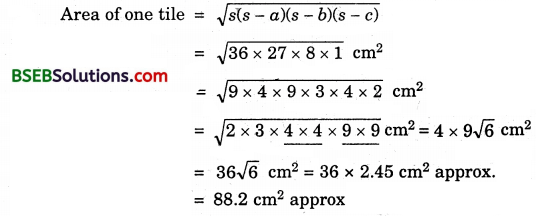∴ Area of 16 such tiles = (16 x 88.2) cm²
= 1411.2 cm² approx.
Cost of polishing @ 50 P per cm² = Rs (1411.2 x 50100)
= Rs 705.60 approx.

Question 9.
A field is in the shape of a trapezium whose parallel sides are 25 m and 10 m. The non-parallel sides are 14 m and 13 m. Find the area of the field.
Solution:
Various lengths are as marked in the figure.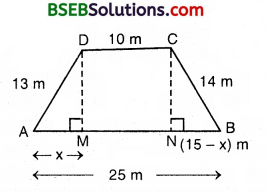Clearly, DM = CN 13 m
⇒ DM² = CN²
⇒ (13)² – x² = 14² – (15 – x)²
⇒ 169 – x² = 196 – (225 – 30x + x²)
⇒ 30x = 169 – 196 + 225 = 198 198
⇒ x = 19830 = 6.6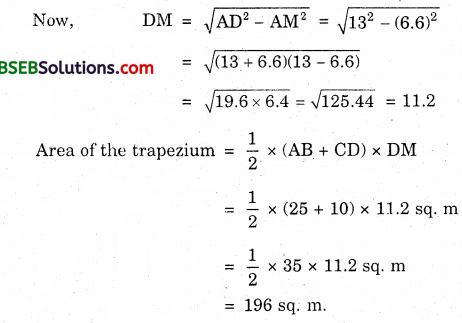## Bihar Board Class 9th Maths Chapter 12 Heron’s Formula Ex 12.2 Textbooks for Exam Preparations

Bihar Board Class 9th Maths Chapter 12 Heron’s Formula Ex 12.2 Textbook Solutions can be of great help in your Bihar Board Class 9th Maths Chapter 12 Heron’s Formula Ex 12.2 exam preparation. The BSEB STD 9th Maths Chapter 12 Heron’s Formula Ex 12.2 Textbooks study material, used with the English medium textbooks, can help you complete the entire Class 9th Maths Chapter 12 Heron’s Formula Ex 12.2 Books State Board syllabus with maximum efficiency.

## FAQs Regarding Bihar Board Class 9th Maths Chapter 12 Heron’s Formula Ex 12.2 Textbook Solutions

#### Can we get a Bihar Board Book PDF for all Classes?

Yes you can get Bihar Board Text Book PDF for all classes using the links provided in the above article.

## Important Terms

Bihar Board Class 9th Maths Chapter 12 Heron’s Formula Ex 12.2, BSEB Class 9th Maths Chapter 12 Heron’s Formula Ex 12.2 Textbooks, Bihar Board Class 9th Maths Chapter 12 Heron’s Formula Ex 12.2, Bihar Board Class 9th Maths Chapter 12 Heron’s Formula Ex 12.2 Textbook solutions, BSEB Class 9th Maths Chapter 12 Heron’s Formula Ex 12.2 Textbooks Solutions, Bihar Board STD 9th Maths Chapter 12 Heron’s Formula Ex 12.2, BSEB STD 9th Maths Chapter 12 Heron’s Formula Ex 12.2 Textbooks, Bihar Board STD 9th Maths Chapter 12 Heron’s Formula Ex 12.2, Bihar Board STD 9th Maths Chapter 12 Heron’s Formula Ex 12.2 Textbook solutions, BSEB STD 9th Maths Chapter 12 Heron’s Formula Ex 12.2 Textbooks Solutions,
Share: